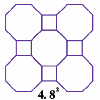#### You may also like### Schlafli Tessellations

are somewhat mundane they do pose a demanding challenge in terms of 'elegant' LOGO procedures. This problem considers the eight semi-regular tessellations which pose a demanding challenge in terms of 'elegant' LOGO procedures.### Napoleon's Theorem

Triangle ABC has equilateral triangles drawn on its edges. Points P, Q and R are the centres of the equilateral triangles. What can you prove about the triangle PQR?

# Polygon Walk

##### Age 16 to 18Challenge Level

Draw the triangle pointing right such that the rightmost vertex is at $\mathbf{i}$

The coordinates of a regular $n$-gon with a centred on the origin with a vertex at $(1,0)$ are

$$\left(\cos\left(\frac{2m\pi}{n}\right), \sin\left(\frac{2m\pi}{n}\right)\right)\, \text{ where }m=0, \dots, n-1$$

For a pentagon, the coordinates become
$$(1, 0), \left(\frac{1}{4}\left(\sqrt{5}-1\right), \frac{1}{4}\left(\sqrt{10+2\sqrt{5}}\right)\right), \left(-\frac{1}{4}\left(\sqrt{5}+1\right), \frac{1}{4}\left(\sqrt{10-2\sqrt{5}}\right)\right)$$
along with the mirror images in the $x$-axis.

This problem builds on GCSE vector work and provides a foundation for concepts met in the later Core A Level modules.